#Convergence of Fourier SeriesMost of the results presented for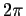-periodic functions extend easily to functions 2L-periodic functions. So we only discuss the case of-periodic functions.

Definition. The function f(x) defined on [a,b], is said to be piecewise continuous if and only if, there exits a partition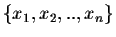of [a,b] such that

(1)
f(x) is continuous on [a,b] except may be for the points xi,
(2)
the right-limit and left-limit of f(x) at the points xi exist.
We will say that f(x) is piecewise smooth if and only if f(x) as well as its derivatives are piecewise continuous.

Recall thatis a partition of [a,b] if

a = x1 < x2 < ..< xn-1 < xn = b.

All the known results on the sum, product, and the quotient are valid for piecewise smooth functions. Except for the fundamental theorem of Calculus which needs to be modified. Indeed, we have let f(x) be piecewise smooth function on the open interval a < x < b. There is no reason for f(x) and f'(x) to be defined at the end-points a and b. But if we denote the left-limit and right-limit of f(x) at a point x0 by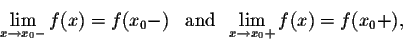the fundamental theorem of Calculus translates into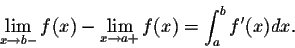Before we state the fundamental result on convergence of Fourier series, we need some intermediary results.

Remark. Recall that our initial problem is to approximate a function globally (on an interval versus Taylor approximations which are local). In this context, the approximation of f(x) will be done via the Fourier polynomials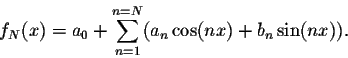These Fourier polynomials will be called the Fourier partial sums.

The fundamental result on convergence of Fourier series, due to Dirichlet, states:

Theorem. Let f(x) be a function, which is twice differentiable, such that f(x), f'(x), and f''(x) are piecewise continuous on the interval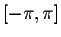. Then, for any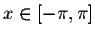, the sequence of Fourier partial sums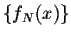converges to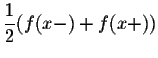, as n tends to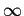.

Recall that the notation f(x+) (resp. f(x-)) represent the right-limit and left-limit respectively of f at the point x. Let us associate to f the new function S(f) defined by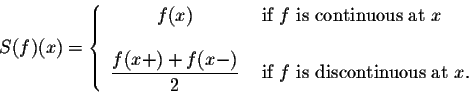The conclusion of the Theorem above translates into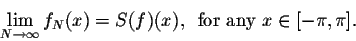Example. Show that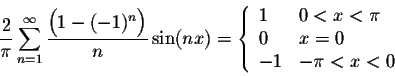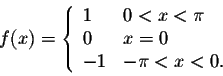This function satisfies the assumptions of the main Theorem. Before, we use the Thoerem's conclusion, let us find its Fourier series. We have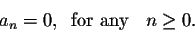Easy calculations give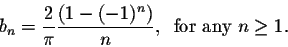The Theorem's conclusion gives the desired identity.

In the next page, you may find more on convergence of Fourier series.[Geometry] [Algebra] [Trigonometry ]
[Calculus] [Differential Equations] [Matrix Algebra]S.O.S MATH: Home Page

Do you need more help? Please post your question on our S.O.S. Mathematics CyberBoard.Author: M.A. Khamsi